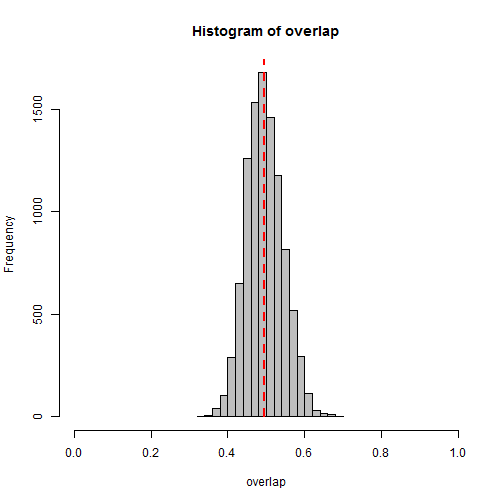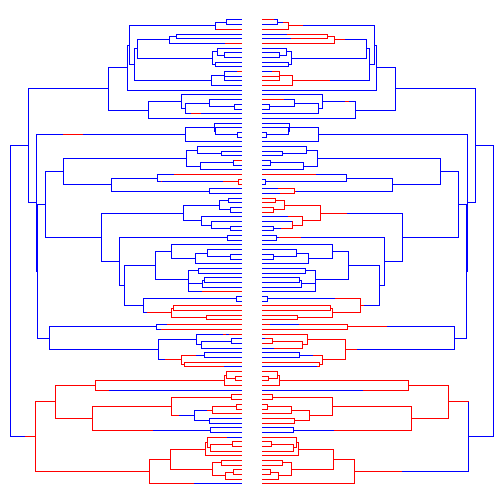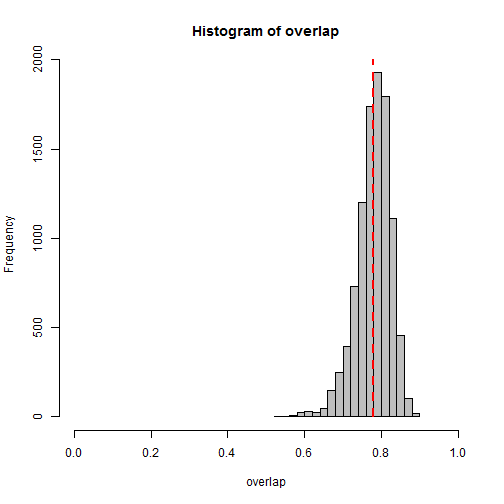## Wednesday, May 20, 2015

### Computing map.overlap for a set of trees

A R-sig-phylo participant recently asked how one goes about using the phytools function `map.overlap` to compute the set of similarities between stochastic mappings for a set of trees. `map.overlap` calculates the summed fraction edge lengths between two trees that are the same. She also wanted to do this if the topologies of different trees from stochastic mapping differed, so long as the 1st tree in set one, and the 1st tree in set two matched, along with the 2nd with the 2nd, and so on.

Well, this is not too challenging. If we have, say, N stochastic mappings on a tree for one character, and N stochastic mappings for a second, and so long as the two characters are coded in the same way (for instance, 0 / 1, A / B, 1 / 2 / 3, etc.), then it is pretty easy to compute the N × N matrix of map overlaps between the two sets:

``````## first let's simulate a tree & two a/b characters to use in
## this demo
library(phytools)
tree<-pbtree(n=100,scale=1)
Q<-matrix(c(-1,1,1,-1),2,2)
rownames(Q)<-colnames(Q)<-letters[1:2]
x<-sim.history(tree,Q)\$states
``````
``````## Done simulation(s).
``````
``````x ## character 1
``````
``````##   t4  t65  t87  t88  t55  t56  t33  t45  t83  t84  t37  t43  t44  t41  t42
##  "b"  "a"  "b"  "b"  "b"  "b"  "b"  "b"  "b"  "b"  "b"  "b"  "b"  "b"  "b"
##  t48  t93  t94  t81  t82   t1  t64  t91  t92  t66  t15  t16  t29  t30  t26
##  "b"  "b"  "b"  "a"  "a"  "b"  "b"  "a"  "a"  "b"  "a"  "a"  "a"  "b"  "a"
##  t79  t80  t21   t5   t6  t34  t35  t10  t11  t95  t96  t19  t28  t31  t32
##  "a"  "a"  "b"  "b"  "a"  "a"  "a"  "b"  "a"  "b"  "b"  "b"  "b"  "b"  "b"
##  t23  t24  t20  t75  t76  t36   t9  t69  t70  t73  t74  t46  t27  t77  t78
##  "b"  "a"  "a"  "a"  "a"  "a"  "b"  "b"  "b"  "a"  "a"  "b"  "b"  "b"  "b"
##  t71  t72  t39  t40  t99 t100   t7  t25  t85  t86  t57  t58  t22  t17  t97
##  "b"  "b"  "a"  "a"  "a"  "a"  "b"  "b"  "b"  "b"  "b"  "b"  "b"  "a"  "a"
##  t98  t50  t49   t3  t18  t89  t90  t38  t14   t2  t47  t62  t63  t59  t51
##  "a"  "a"  "b"  "a"  "b"  "b"  "b"  "b"  "b"  "b"  "b"  "b"  "b"  "b"  "a"
##  t52  t60  t61  t54   t8  t12  t13  t53  t67  t68
##  "a"  "a"  "a"  "b"  "b"  "b"  "b"  "b"  "b"  "b"
``````
``````y<-sim.history(tree,Q)\$states
``````
``````## Done simulation(s).
``````
``````y ## character 2
``````
``````##   t4  t65  t87  t88  t55  t56  t33  t45  t83  t84  t37  t43  t44  t41  t42
##  "a"  "b"  "b"  "b"  "b"  "b"  "a"  "a"  "a"  "a"  "a"  "a"  "a"  "b"  "b"
##  t48  t93  t94  t81  t82   t1  t64  t91  t92  t66  t15  t16  t29  t30  t26
##  "b"  "b"  "b"  "a"  "a"  "b"  "a"  "a"  "a"  "a"  "b"  "a"  "a"  "a"  "b"
##  t79  t80  t21   t5   t6  t34  t35  t10  t11  t95  t96  t19  t28  t31  t32
##  "b"  "b"  "b"  "b"  "b"  "a"  "a"  "b"  "a"  "b"  "b"  "b"  "b"  "b"  "b"
##  t23  t24  t20  t75  t76  t36   t9  t69  t70  t73  t74  t46  t27  t77  t78
##  "b"  "b"  "b"  "a"  "a"  "a"  "b"  "b"  "b"  "b"  "b"  "b"  "b"  "b"  "b"
##  t71  t72  t39  t40  t99 t100   t7  t25  t85  t86  t57  t58  t22  t17  t97
##  "b"  "b"  "a"  "a"  "a"  "a"  "a"  "a"  "a"  "a"  "a"  "a"  "b"  "a"  "b"
##  t98  t50  t49   t3  t18  t89  t90  t38  t14   t2  t47  t62  t63  t59  t51
##  "b"  "b"  "b"  "a"  "a"  "a"  "a"  "a"  "a"  "a"  "a"  "a"  "a"  "a"  "a"
##  t52  t60  t61  t54   t8  t12  t13  t53  t67  t68
##  "a"  "a"  "a"  "a"  "a"  "a"  "b"  "b"  "b"  "b"
``````
``````## next we can generate a set of 100 stochastic maps for each
## character
mx<-make.simmap(tree,x,nsim=100)
``````
``````## make.simmap is sampling character histories conditioned on the transition matrix
## Q =
``````
``````##           a         b
## a -1.070102  1.070102
## b  1.070102 -1.070102
``````
``````## (estimated using likelihood);
## and (mean) root node prior probabilities
## pi =
``````
``````##   a   b
## 0.5 0.5
``````
``````## Done.
``````
``````my<-make.simmap(tree,y,nsim=100)
``````
``````## make.simmap is sampling character histories conditioned on the transition matrix
## Q =
``````
``````##            a          b
## a -0.7767663  0.7767663
## b  0.7767663 -0.7767663
``````
``````## (estimated using likelihood);
## and (mean) root node prior probabilities
## pi =
``````
``````##   a   b
## 0.5 0.5
``````
``````## Done.
``````
``````## compute the matrix of overlaps
overlap<-sapply(mx,function(x,y) sapply(y,map.overlap,x),y=my)
dim(overlap)
``````
``````##  100 100
``````
``````mean(overlap)
``````
``````##  0.495982
``````
``````hist(overlap,xlim=c(0,1),col="grey")
lines(c(mean(overlap),mean(overlap)),c(0,par()\$usr),lty="dashed",
col="red",lwd=2)
``````Note that this matrix is assymetric as `overlap[i,j]` gives the map similarity between `mx[[i]]` and `my[[j]]`, in this case.

Alternatively, if we want to compute the vector of pairwise comparison, 1 vs. 1, 2 vs. 2, 3 vs. 3, and so on, we can do that using `mapply`:

``````overlap<-mapply(map.overlap,mx,my)
length(overlap)
``````
``````##  100
``````
``````mean(overlap)
``````
``````##  0.4902131
``````

Now, a separate question might be what the stochastic mapping similarities of two different characters might tell us about a correlation, or lack thereof, in their evolution process. To examine this, I thought I'd use some code that I posted earlier to simulate correlated evolution of two binary traits:

``````## values of Q to on average produce the same rates
## in x & y as before
Q<-matrix(c(0,0.8,0.8,0,3.2,0,0,3.2,3.2,0,0,3.2,0,0.8,0.8,0),4,4,byrow=TRUE)
rownames(Q)<-colnames(Q)<-c("aa","ab","ba","bb")
diag(Q)<--rowSums(Q)
tt<-sim.history(tree,t(Q))
``````
``````## Note - the rate of substitution from i->j should be given by Q[j,i].
## Done simulation(s).
``````
``````tt1<-mergeMappedStates(tt,c("aa","ab"),"a")
tt1<-mergeMappedStates(tt1,c("ba","bb"),"b")
tt2<-mergeMappedStates(tt,c("aa","ba"),"a")
tt2<-mergeMappedStates(tt2,c("ab","bb"),"b")
## these data are correlated, see:
par(mfrow=c(1,2))
plotSimmap(tt1,setNames(c("blue","red"),letters[1:2]),ftype="off",lwd=1)
plotSimmap(tt2,setNames(c("blue","red"),letters[1:2]),ftype="off",lwd=1,
direction="leftwards")
````````````## pull the tip states out of our simulated histories
x<-getStates(tt1,"tips")
y<-getStates(tt2,"tips")
``````

Now let's do stochastic mapping for both characters and then compute the mean & distribution of overlap in the stochastic. Obviously, as the characters are correlated, we might expect that they show a higher degree of overlap in the stochastic maps.

``````mx<-make.simmap(tree,x,nsim=100)
``````
``````## make.simmap is sampling character histories conditioned on the transition matrix
## Q =
``````
``````##            a          b
## a -0.8040389  0.8040389
## b  0.8040389 -0.8040389
``````
``````## (estimated using likelihood);
## and (mean) root node prior probabilities
## pi =
``````
``````##   a   b
## 0.5 0.5
``````
``````## Done.
``````
``````my<-make.simmap(tree,y,nsim=100)
``````
``````## make.simmap is sampling character histories conditioned on the transition matrix
## Q =
``````
``````##            a          b
## a -0.9141153  0.9141153
## b  0.9141153 -0.9141153
``````
``````## (estimated using likelihood);
## and (mean) root node prior probabilities
## pi =
``````
``````##   a   b
## 0.5 0.5
``````
``````## Done.
``````
``````overlap<-sapply(mx,function(x,y) sapply(y,map.overlap,x),y=my)
mean(overlap)
``````
``````##  0.7793841
``````
``````hist(overlap,xlim=c(0,1),col="grey")
lines(c(mean(overlap),mean(overlap)),c(0,par()\$usr),lty="dashed",
col="red",lwd=2)
``````Interesting.

Now, does this tell us anything that Pagel's (1994) method (implemented now in the phytools function `fitPagel` wouldn't have - I don't know, but it is kind of neat to see in any case.

That's all for now.